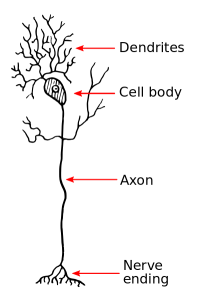An artificial neural net is electrically analogous to of a biological neural net. A biological neural net is a collection of nerve fibres, or “neurons”. A neuron is the structural and functional unit of the human nervous sytem, which is active in receiving and transmitting electrical signals after processing them, to and from its neighbors. It is believed that the neuron converts the received signal amplitudes into a weighted average, and through a limiting non-linear function, further propagates the processed resultant signal.

A neuron’s main structural components are the cell body, the dendrites, axon and synapse (axon terminals), as shown below:Fig 1. A Simple Biological Neuron Structure

Fig 2. Artificial Neural Net

Here, x(i) is the ith input signal, and w(i) is the “weight”, associated with the input. The weight is the quantity that may get updated in a number of iterations in the learning process, such that the neural net works more and more in the fashion of the required system model, producing more suitable outputs for a set of inputs.

The artificial neural net attempts to follow the biological neuron, by modeling its response characteristics using an enhancing activation function (f(s)), similar to conduction of a signal through a resistance. The synapse of the neuron is imitated by a non-linear limiting function which performs amplitude limitation.

The net can be implemented using a mathematical Sigmoid function, where output is given as:

output=1/(1+exp(-net))

Also, by other functions such as the tanh function, signum and step functions.

Many different topologies may be defined in the network, commonly the single-layered net, two-layered feed-forward structure or feedback structure, three-layered feed-forward, etc.

There are primarily three kinds of machine learning associated with the neural networks, namely, Supervised, Unsupervised and Reinforcement Learning.

## Supervised Learning

In the Supervised algorithm, both the input and required output are known a priori, which means that the learning is based on knowing and minimizing the error involved between the desired output and the actual output. An example of Supervised learning is the Back-propagation algorithm. Thus the method works on the basis of weight adjustment through error measure feedback.

## Unsupervised Learning

In Unsupervised kind of learning, the required output is unknown. The descriptor inputs are each assigned to a “node”, or class representing similarity of outputs. The job of the learning process is to adjust the weights by an autonomous function, independent of a known target output, in order to classify the inputs according to the recursively updating weights. The recursive relation may be formulated depending on the topoplogy and application, for example, Hopfield net, cognitive neural nets, etc.

## Reinforncement Learning

Reinforcement Learning is the type of learning which falls in between the other two types. Here, the learning is based on a reaction to an action, i.e, a “reward” or a “punishment” towards the action. By this, the parameters may be adjusted by subsequently increasing or decreasing, or other similar changes in accordance to the reaction measure. This is continued until equilibrium is reached, such that no further changes are detected in the parameters. Self organizing learning is categorized in this.

Share.